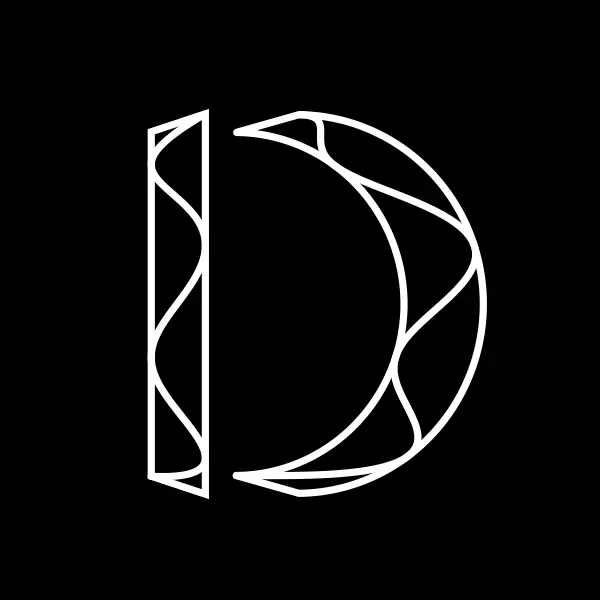# Fine Design

A YouTube channel featuring some maths and theoretical computer science videos.What does this logo even represent?

Fine Design (turns out you can’t change the name of a channel once you create it) was a result of me trying out Grant Sanderson’s amazing manim library for mathematics-related animations. Thanks to that I ended up making a bunch of videos about topics that I considered layman-accessible but that I couldn’t find on YouTube. Here’s a list of topics covered.

• A couple of hat puzzles that I really liked for their basic yet insightful impossibility proofs, and for the impressive possibility results that follow.

• A series on the BIGNUM BAKEOFF contest, where contestants had to submit $512$-character C codes and the code that returns the largest number (when run on a hypothetical computer with arbitrarily large ints) wins. It touches a lot of topics in theoretical computer science and mathematical logic.

• A video on non-adaptive combinatorial group testing. It goes over how polynomials were used by some researchers to test people for covid with far fewer tests performed than the number of people tested.

• A video on the Graham-Pollak Theorem and how the proof of such a combinatorial statement is so “non-combinatorial”.

• A series on Markov’s Theorem (the one that bounds the maximum slope of a degree $d$ polynomial that is bounded in an interval). There’s a fascinating proof that I’ve tried to present.

• A series on quantum brute-force password checking, with an upper bound from Grover’s algorithm and a matching lower bound from Markov’s theorem.

• If you’re allowed to change a number $N$ in base $10$ by adding plus signs in the middle, (i.e. $15243 \to 1+5+24+3 = 32$ is a valid step), then as $N \to \infty$, how many steps would you need to reach a single-digit number? $O(\log^*N)$ is easy to prove, but can you show a better upper bound?##### Suhail Sherif
###### Postdoctoral Researcher

I work in theoretical computer science, with a focus on query and communication complexity.